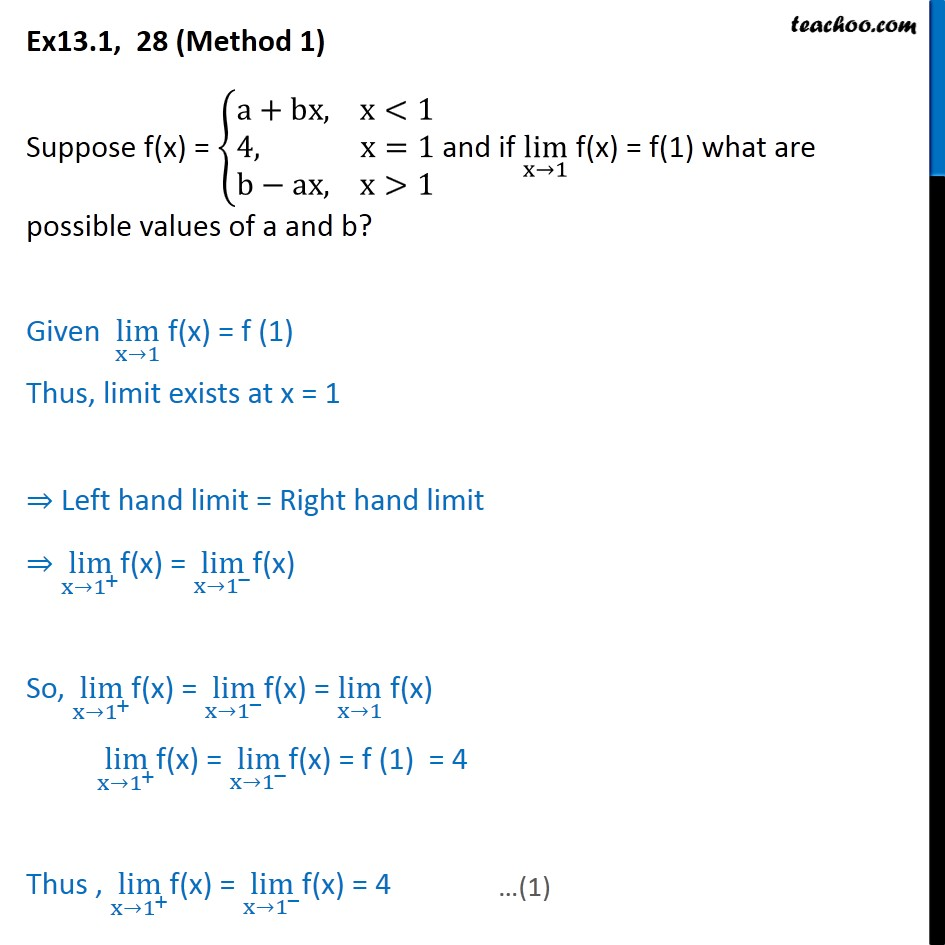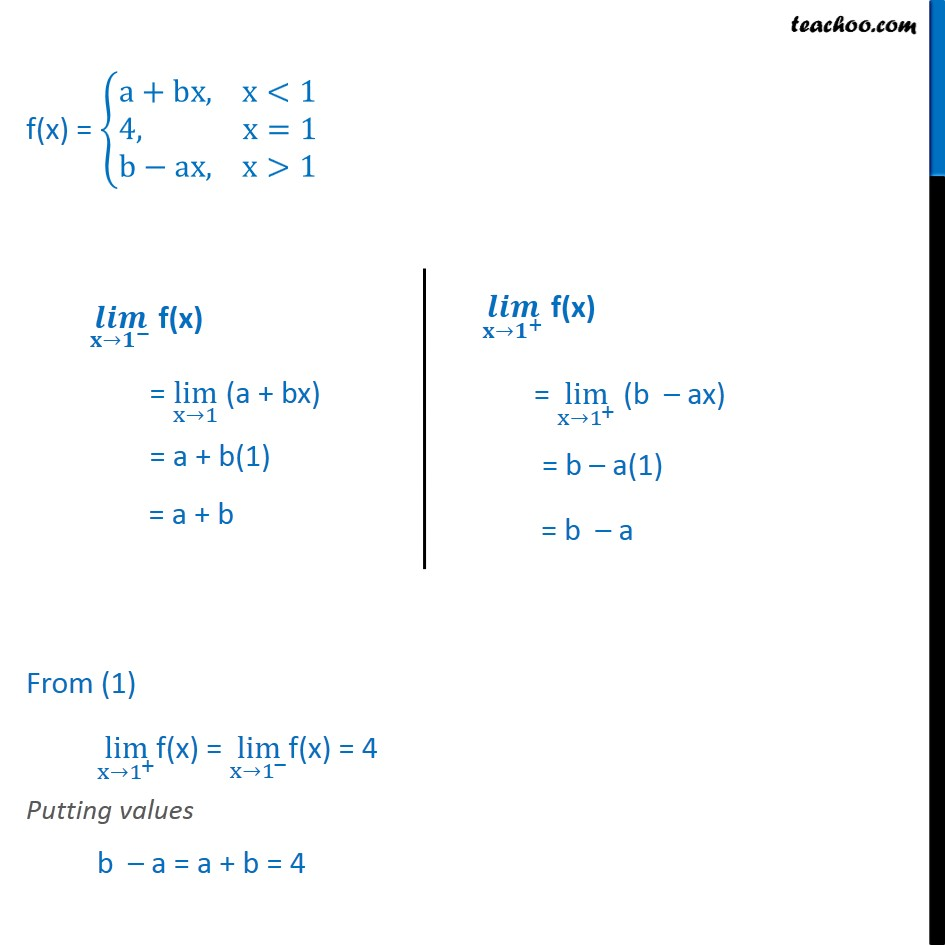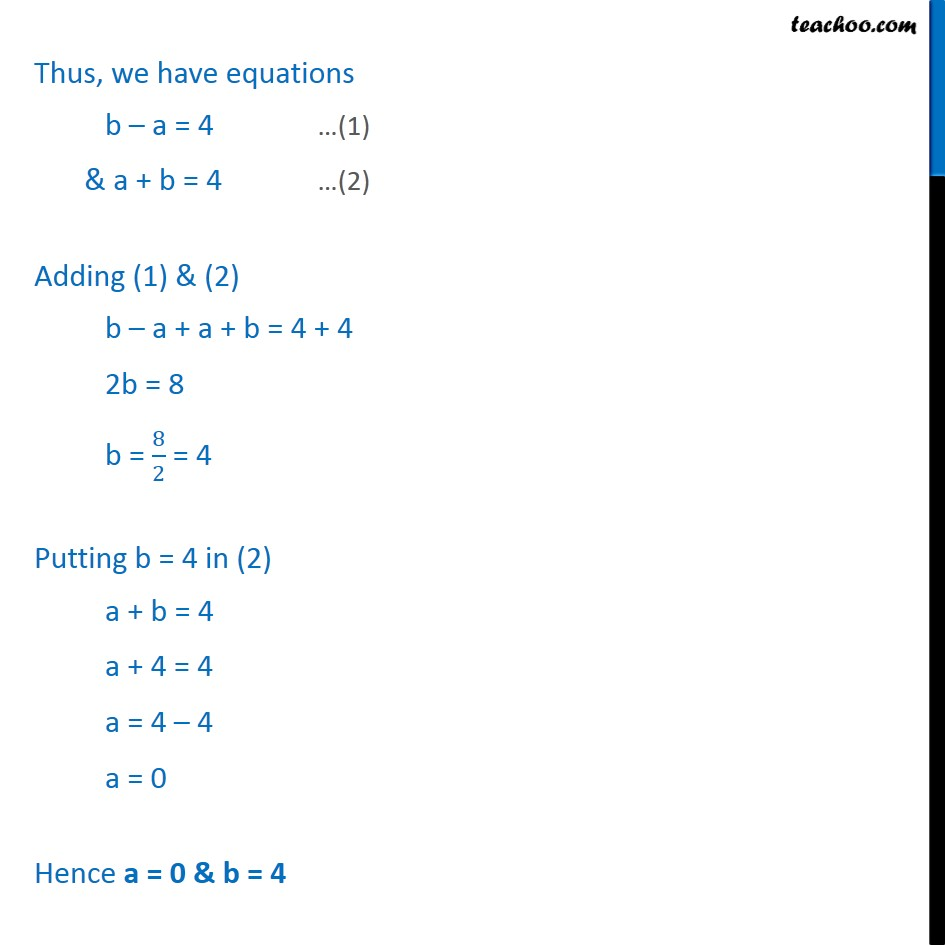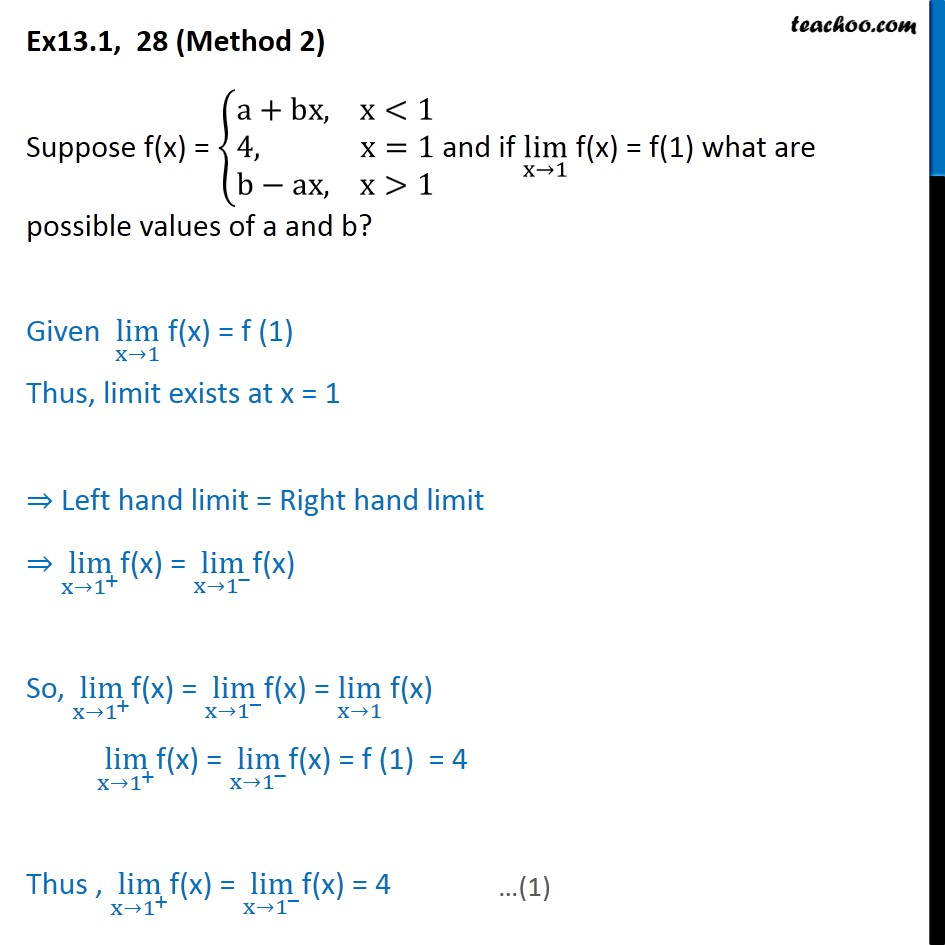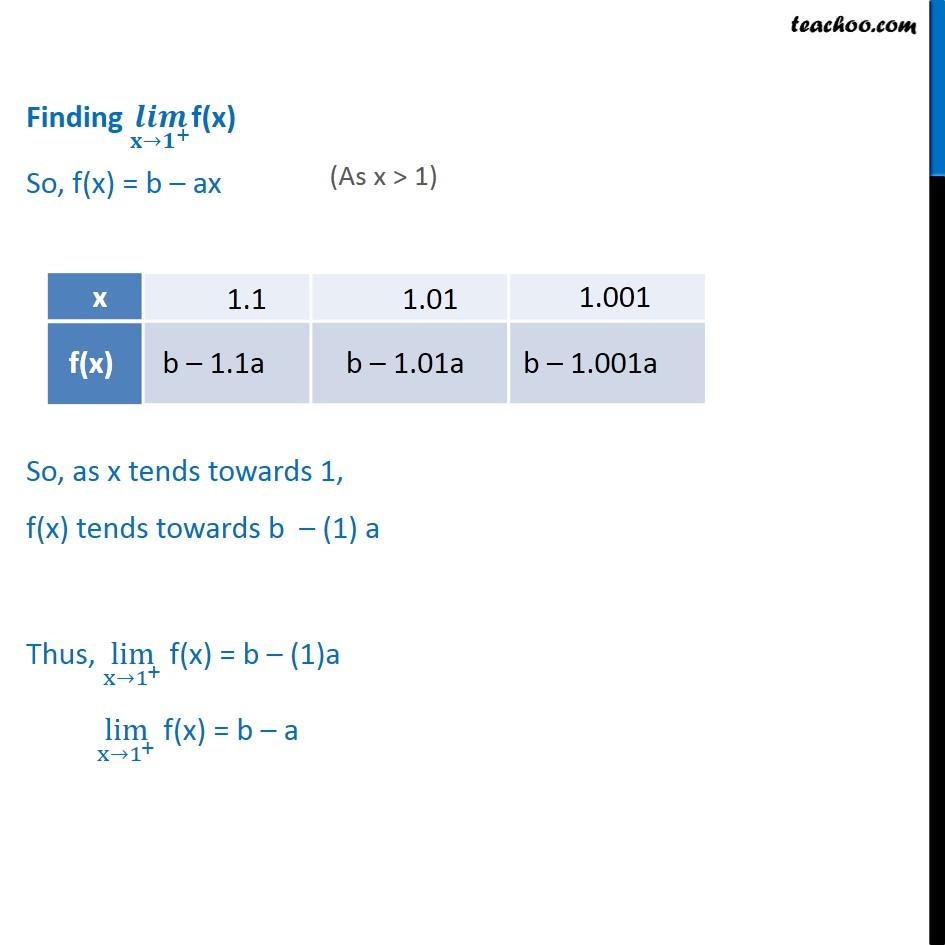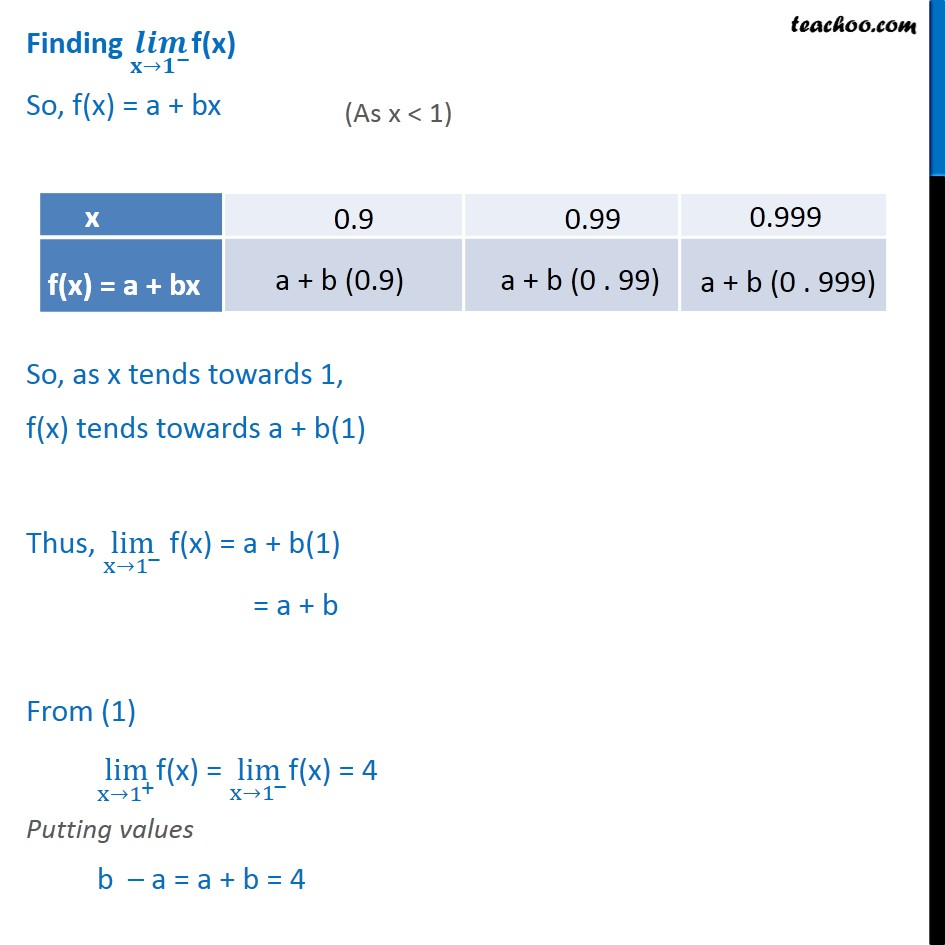1. Class 11
2. Important Question for exams Class 11
3. Chapter 13 Class 11 Limits and Derivatives

Transcript

Ex 13.1, 28 (Method 1) Suppose f(x) = a+bx, x<1 4, x=1 b ax, x>1 and if lim x 1 f(x) = f(1) what are possible values of a and b? Given lim x 1 f(x) = f (1) Thus, limit exists at x = 1 Left hand limit = Right hand limit lim x 1 + f(x) = lim x 1 f(x) So, lim x 1 + f(x) = lim x 1 f(x) = lim x 1 f(x) lim x 1 + f(x) = lim x 1 f(x) = f (1) = 4 Thus , lim x 1 + f(x) = lim x 1 f(x) = 4 f(x) = a+bx, x<1 4, x=1 b ax, x>1 From (1) lim x 1 + f(x) = lim x 1 f(x) = 4 Putting values b a = a + b = 4 Thus, we have equations b a = 4 (1) & a + b = 4 (2) Adding (1) & (2) b a + a + b = 4 + 4 2b = 8 b = 8 2 = 4 Putting b = 4 in (2) a + b = 4 a + 4 = 4 a = 4 4 a = 0 Hence a = 0 & b = 4 Ex13.1, 28 (Method 2) Suppose f(x) = a+bx, x<1 4, x=1 b ax, x>1 and if lim x 1 f(x) = f(1) what are possible values of a and b? Given lim x 1 f(x) = f (1) Thus, limit exists at x = 1 Left hand limit = Right hand limit lim x 1 + f(x) = lim x 1 f(x) So, lim x 1 + f(x) = lim x 1 f(x) = lim x 1 f(x) lim x 1 + f(x) = lim x 1 f(x) = f (1) = 4 Thus , lim x 1 + f(x) = lim x 1 f(x) = 4 Finding + f(x) So, f(x) = b ax So, as x tends towards 1, f(x) tends towards b (1) a Thus, lim x 1 + f(x) = b (1)a lim x 1 + f(x) = b a Finding f(x) So, f(x) = a + bx So, as x tends towards 1, f(x) tends towards a + b(1) Thus, lim x 1 f(x) = a + b(1) = a + b From (1) lim x 1 + f(x) = lim x 1 f(x) = 4 Putting values b a = a + b = 4 Thus, we have equations b a = 4 (1) & a + b = 4 (2) Adding (1) & (2) b a + a + b = 4 + 4 2b = 8 b = 8 2 = 4 Putting b = 4 in (2) a + b = 4 a + 4 = 4 a = 4 4 a = 0 Hence a = 0 & b = 4

Chapter 13 Class 11 Limits and Derivatives

Class 11
Important Question for exams Class 11

About the AuthorDavneet Singh
Davneet Singh is a graduate from Indian Institute of Technology, Kanpur. He has been teaching from the past 9 years. He provides courses for Maths and Science at Teachoo.